Publicité

# Types of Interest.pptx

22 Mar 2023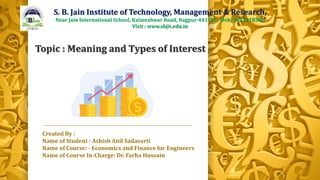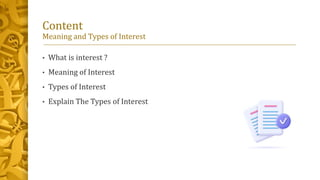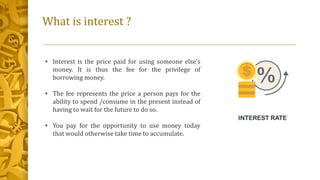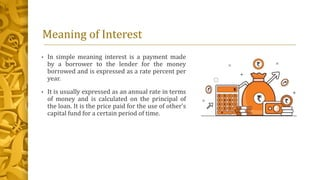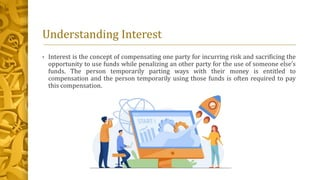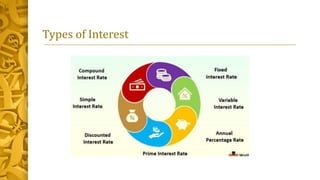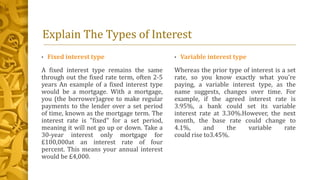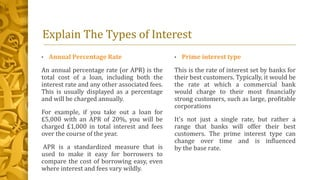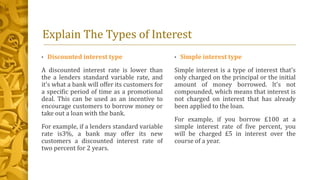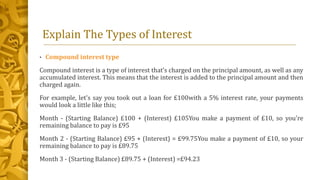1 sur 11
Publicité

### Types of Interest.pptx

1. Created By : Name of Student : Ashish Anil Sadavarti Name of Course: - Economics and Finance for Engineers Name of Course In-Charge: Dr. Farha Hussain S. B. Jain Institute of Technology, Management & Research, Near Jain International School, Kalmeshwar Road, Nagpur-441501. Mob. 9823218380 Visit : www.sbjit.edu.in Topic : Meaning and Types of Interest
2. Content Meaning and Types of Interest • What is interest ? • Meaning of Interest • Types of Interest • Explain The Types of Interest
3. What is interest ? • Interest is the price paid for using someone else's money. It is thus the fee for the privilege of borrowing money. • The fee represents the price a person pays for the ability to spend /consume in the present instead of having to wait for the future to do so. • You pay for the opportunity to use money today that would otherwise take time to accumulate.
4. Meaning of Interest • In simple meaning interest is a payment made by a borrower to the lender for the money borrowed and is expressed as a rate percent per year. • It is usually expressed as an annual rate in terms of money and is calculated on the principal of the loan. It is the price paid for the use of other’s capital fund for a certain period of time.
5. Understanding Interest • Interest is the concept of compensating one party for incurring risk and sacrificing the opportunity to use funds while penalizing an other party for the use of someone else’s funds. The person temporarily parting ways with their money is entitled to compensation and the person temporarily using those funds is often required to pay this compensation.
6. Types of Interest
7. Explain The Types of Interest • Fixed interest type A fixed interest type remains the same through out the fixed rate term, often 2-5 years An example of a fixed interest type would be a mortgage. With a mortgage, you (the borrower)agree to make regular payments to the lender over a set period of time, known as the mortgage term. The interest rate is "fixed" for a set period, meaning it will not go up or down. Take a 30-year interest only mortgage for £100,000at an interest rate of four percent. This means your annual interest would be £4,000. • Variable interest type Whereas the prior type of interest is a set rate, so you know exactly what you're paying, a variable interest type, as the name suggests, changes over time. For example, if the agreed interest rate is 3.95%, a bank could set its variable interest rate at 3.30%.However, the next month, the base rate could change to 4.1%, and the variable rate could rise to3.45%.
8. Explain The Types of Interest • Annual Percentage Rate An annual percentage rate (or APR) is the total cost of a loan, including both the interest rate and any other associated fees. This is usually displayed as a percentage and will be charged annually. For example, if you take out a loan for £5,000 with an APR of 20%, you will be charged £1,000 in total interest and fees over the course of the year. APR is a standardized measure that is used to make it easy for borrowers to compare the cost of borrowing easy, even where interest and fees vary wildly. • Prime interest type This is the rate of interest set by banks for their best customers. Typically, it would be the rate at which a commercial bank would charge to their most financially strong customers, such as large, profitable corporations It's not just a single rate, but rather a range that banks will offer their best customers. The prime interest type can change over time and is influenced by the base rate.
9. Explain The Types of Interest • Discounted interest type A discounted interest rate is lower than the a lenders standard variable rate, and it's what a bank will offer its customers for a specific period of time as a promotional deal. This can be used as an incentive to encourage customers to borrow money or take out a loan with the bank. For example, if a lenders standard variable rate is3%, a bank may offer its new customers a discounted interest rate of two percent for 2 years. • Simple interest type Simple interest is a type of interest that's only charged on the principal or the initial amount of money borrowed. lt's not compounded, which means that interest is not charged on interest that has already been applied to the loan. For example, if you borrow £100 at a simple interest rate of five percent, you will be charged £5 in interest over the course of a year.
10. Explain The Types of Interest • Compound interest type Compound interest is a type of interest that’s charged on the principal amount, as well as any accumulated interest. This means that the interest is added to the principal amount and then charged again. For example, let's say you took out a loan for £100with a 5% interest rate, your payments would look a little like this; Month - (Starting Balance) £100 + (Interest) £105You make a payment of £10, so you're remaining balance to pay is £95 Month 2 - (Starting Balance) £95 + (Interest) = £99.75You make a payment of £10, so your remaining balance to pay is £89.75 Month 3 - (Starting Balance) £89.75 + (Interest) =£94.23
Publicité